# Vocabulary-Number Sense Flashcards

Set Details Share
created 12 years ago by fmarlatt
1,156 views
updated 11 years ago by fmarlatt
Page to share:
Embed this setcancel
COPY
code changes based on your size selection
Size:
X

1

Commutative Property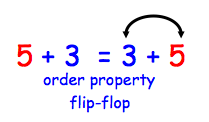2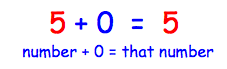3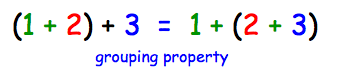4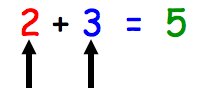5

sum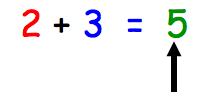6

round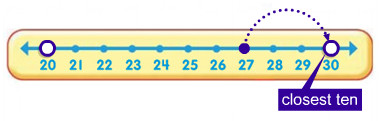ABOUT how many or how much.
Closest number ending with 0.

7

estimateTo give a number or answer that tells about how many or how much.

8

compatible numbers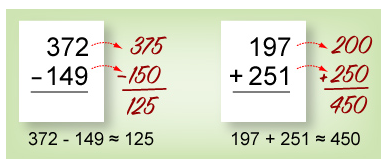Numbers that are easy to add, subtract, multiply, or divide mentally.

9

fact family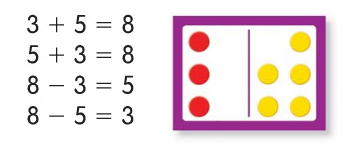a group of related facts using the same numbers

10

difference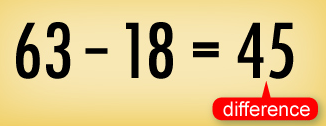The answer to a subtraction problem.

11

equivalent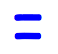equal to

12

compute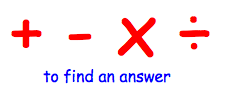13

expanded form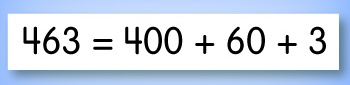14

inverse operations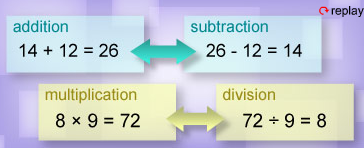Two operations that are the opposite of each other.

15

compensation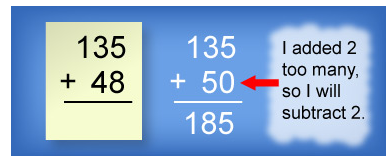adding and subtracting the same number to make the sum or difference easier to find

16

numerator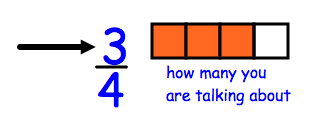17

standard form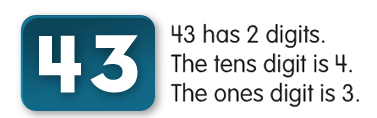a way to write a number only showing its digits
Example: 385

18

denominator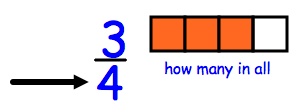19

word form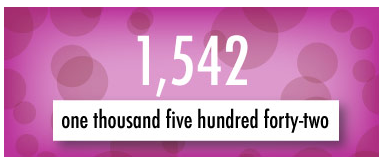a number written in words

four thousand, six hundred, thirty two

20

base 10 place value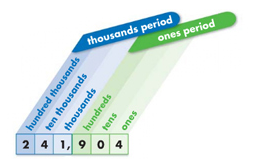the value of the place a digit has in a number

21

digits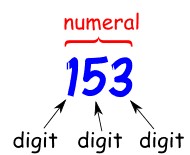the symbols 0, 1, 2, 3, 4, 5, 6, 7, 8,and 9, used to write numbers

22

compare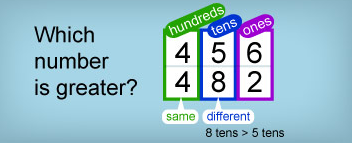to find out which number is greater and which number is less

23

ordinal number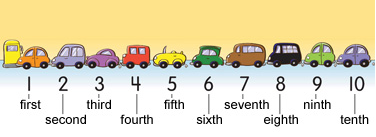a number used to show the order of people or objects

24

decimal point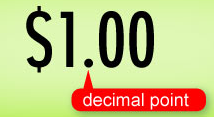a dot that separates dollars from cents and ones from tenths in a number
Example: \$1.95, 1.9

25

integers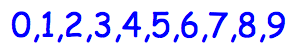26

array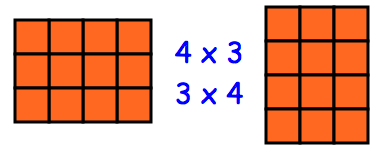27

skip count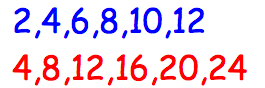28

set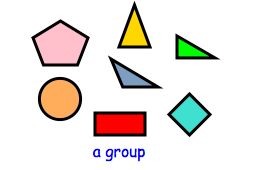29

subset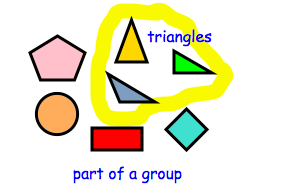30

whole numbers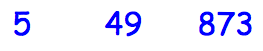no fraction, no decimals, no negatives

31

at least

as much as, equal to or greater than

32

at most

no more than, equal to or less than

33

factor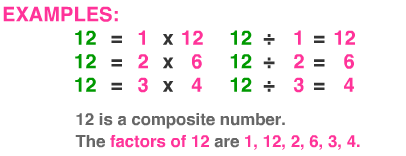a whole number that multiplies with another number to make a third number

34

fraction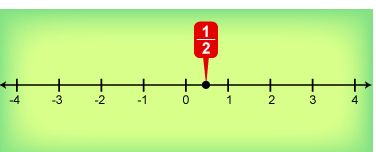any part of a group, number or whole

Fractions have a numerator and a denominator

35

greater than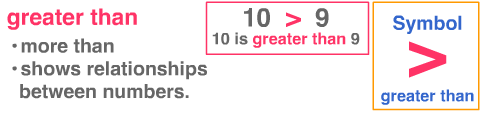36

less than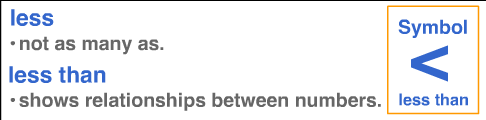37

mixed number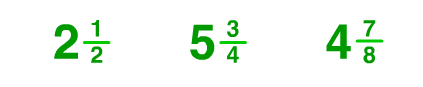a whole number and a fraction

38

model

...

39

multiplication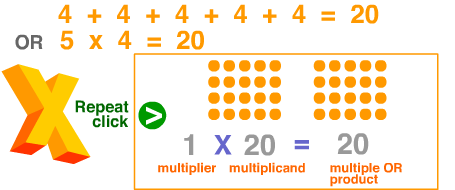an mathematical operation where a number is added to itself several times

40

division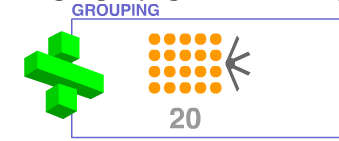a math operation where a number is shared or grouped into equal parts

41

a math operation where 2 or more groups are combined

45 + 13 = 58

42

subtraction

a math operation that takes one quantity away from another

63 - 19 = 44

43

number sentence

a mathematical sentence using symbols and numbers

an equation or a inequality with <, >, or =

2 + 3 = 5
1 + 3 > 2
8 - 2 < 7

44

place value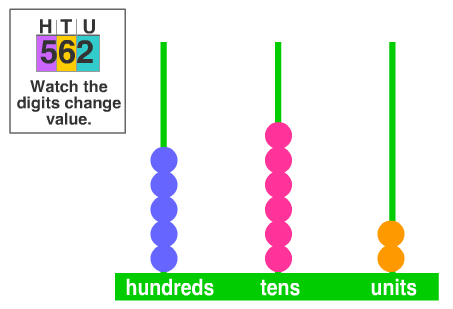the value of a digit depending on its place in a number

45

reasonable

46

regrouping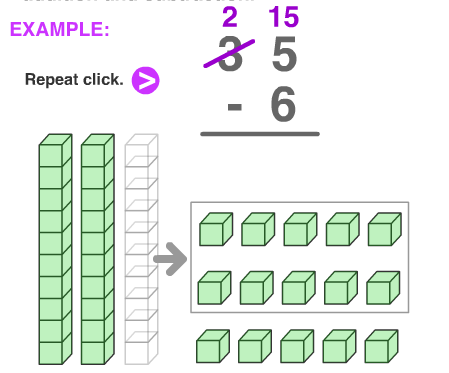carrying in addition and borrowing in subtraction

47

sharing equally

fair share, dividing so that each group is equal

48

classify

to make a group based on similar characteristics

49

orderarranged according to size, amount or value

50

distributive property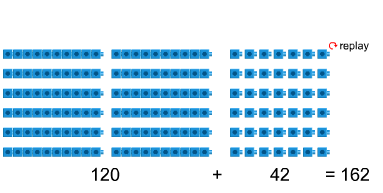6 x 27 =

breaking apart multiplication problems into similar problems